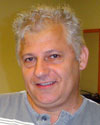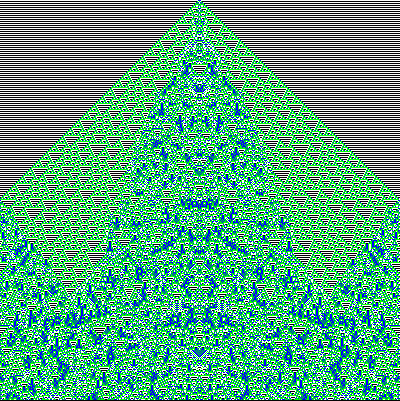#Wolfram Summer School

# Alumni# Alexandru Lupascu

Summer School

Class of 2005

## Bio

Alexandru Lupascu graduated as a physicist-engineer from the Electronic Faculty, Polytechnic University Bucharest (Romania). In 1984 he obtained his Ph.D. in physics from the same university, working in lasers and atomic spectroscopy. He used functions of a matrix to represent atomic collisions. During this period he became interested in quantum mechanics and put into operation the same mathematical method for the time evolution of quantum systems. In 1992-1996 he accomplished several stages in Grenoble, working at GeeO, an integrated optics laboratory associated with INPG and Schneider. He studied rare-earth spectroscopy in glasses and did computer simulations in Mathematica for ionic interdiffusion as a tool to produce optical wave-guides. He solved nonlinear diffusion equations with concentration-dependent coefficients and observed the role of local charge density during field-assisted processes. He is currently working in three domains, all employing Mathematica programming: minimal conditions for the way a classical system would acquire quantum characters, computations for differential geometry problems, and Fourier analysis of non-linear dynamic processes. He spent his career at the physics department of the Polytechnic University Bucharest, where he presently is a professor. He teaches lectures in general physics, statistical physics, integrated optics, and laser applications.

## Project: Cellular Automata Approximating the Equations of Optics

Optics is described by wave equations. Their solutions may superpose, giving interference and diffraction maxima and minima. One tries to represent these characteristic fringes by CAs. The figures must present clear wavy patterns. When initial conditions introduce more than one source, interference and/or diffraction are present and must be seen. Other apparent optical features have to be described. Electromagnetic waves are often described by complex quantities varying with position and time. Hence we can represent either the modulus or the phase of the field. The phase varies continuously between 0 and 2, and a discrete CA needs only a finite set of colors. Hence the phase must be approximated. An obvious choice is to use only five different values, namely 0, 1, I, -1, -I. The clearest feature of a wave pattern is given by its surfaces of constant phase, so we choose to represent these surfaces.

Considering the time for computation, we have worked only with 3×3 and 4×4 kernels. Certain types of kernels are more efficient in emulating directional propagation. Among the promising CAs the most interesting is 1240.

## Favorite Four-Color, Nearest-Neighbor, Totalistic Rule

Rule chosen: 121307

It reveals much, but may also hide a lot.APPLY NOW